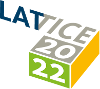#The 39th International Symposium on Lattice Field Theory (Lattice 2022)

Aug 8 – 13, 2022
Hörsaalzentrum Poppelsdorf
Europe/Berlin timezone

## Neutrinoless Double Beta Decay from Lattice QCD: The Short-Distance $\pi^-\rightarrow\pi^+ e^- e^-$ Amplitude

Aug 12, 2022, 2:50 PM
20m
CP1-HSZ/0.001 (CP1-HSZ) - HS3 (CP1-HSZ)

### CP1-HSZ/0.001 (CP1-HSZ) - HS3

#### CP1-HSZ

50
Show room on map
Oral Presentation QCD in searches for physics beyond the Standard Model

### Speaker

Patrick Oare (MIT)

### Description

This talk presents a determination of the short-distance contributions to the unphysical $\pi^-\rightarrow\pi^+ e^- e^-$ decay through lattice QCD calculations. The hadronic contributions to the transition amplitude are described by the pion matrix elements of five Standard Model Effective Field Theory operators, which are computed on five ensembles of domain-wall fermions with $N_f = 2 + 1$ quark flavors with a range of heavier-than-physical values of the light quark mass. The matrix elements are extrapolated to the continuum limit and to the physical light quark mass and infinite volume limit using a functional form derived in chiral perturbation theory. This extrapolation also yields the pion-pion low-energy constants of chiral Effective Field Theory ($\chi\mathrm{EFT}$), which are necessary input for $\chi\mathrm{EFT}$ calculations of neutrinoless double beta decay in nuclei.

### Primary authors

Dr David Murphy (Massachusetts Institute of Technology) William Detmold (MIT) William Jay (Massachusetts Institute of Technology) Patrick Oare (MIT) Phiala Shanahan (MIT)